: Mathematical Theory of the Josephus Problem. : The Self-Similarity of the Josephus Problems. : Linear Josephus Problem.

# The Linear Josephus Problem in Both Directions.

This is the linear Josephus problem in both directions. This variant has been introduced by the authors, but they have not published it in any mathematical journal.

The Self-Similarity of the Josephus Problems

Definition 4.1   Letandbe natural numbers such that. We putnumbers on a line. two numbers are to be removed at the same time, but two processes of elimination go for different directions. Suppose that there are-numbers. Then the first process of elimination starts counting up from the first number and remove the-th,-th,-th number, ... . The second process counts down from the-th number, and the-th,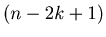-th,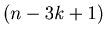-th number, ... are to be removed. We suppose that the first process comes first and the second process second at every stage. We change the direction when we reach the end of the line. Then we begin removing everyth number again.
We call this the linear Josephus Problem in both directions. We denote the position of the survivor by.

Example 4.1   This is a Mathematica program to calculate. The following Mathematica functionreturns the value of.
```linearinter[n_, h_] :=
Block[{p, q, u, rs}, rs = h - 1; elim = {}; elim2 = {};
p = Join[Range[n], Sort[Table[m, {m, 2, n - 1}], Greater]];
q = Reverse[Join[Reverse[Range[n]], Table[k, {k, 2, n - 1}]]];
Do[Do[u1 = First[p];
u2 = First[RotateLeft[p, 1]];
If[u1 == u2, p = RotateLeft[p, 2], p = RotateLeft[p, 1]],
{s, 1, rs}];
u = First[p];
p = Rest[p]; elim = Append[elim, u];
If[MemberQ[p, u], p = Delete[p, Position[p, u]],];
If[MemberQ[q, u], q = Delete[q, Position[q, u]],];
If[Length[Union[p]] == 1, Break[],];
Do[u1 = Last[q];
u2 = Last[RotateRight[q, 1]];
If[u1 == u2, q = RotateRight[q, 2], q = RotateRight[q, 1]],
{s,1, rs}];
u = Last[q];
q = Drop[q, -1]; elim2 = Append[elim2, u];
If[MemberQ[q, u], q = Delete[q, Position[q, u]],];
If[MemberQ[p, u], p = Delete[p, Position[p, u]],];
If[Length[Union[q]] == 1, Break[],], {t, 1, Ceiling[n/2]}];
p[]];
```

By using the Mathematica function in Example 4.1 we can make the graph of the list {JLI(n,k), n = 1,2,3,... }.

Example 4.2   Graph 4.1 and Graph 4.2 are the graphs of the lists {, n = 1,2,..,1024} and {, n = 1,2,..,256}. Please compare these two graphs．They have the same shape, and hence these graphs seem to have Fractal behavior (self-similarity). Note that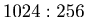is approximately equal to.

Graph 4.1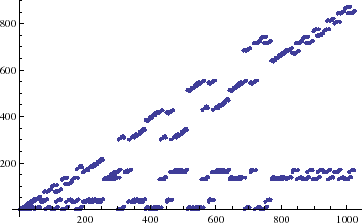Graph 4.2We are going to prove that the graph ofin Example 4.2 has the self-similarity with the ratio ofin the next section.

The authors have not proved the existence of the self-similarity for. As to these problems the authors are going to present only graphs, and the graphs seem to have the self-similarity.

Example 4.3   Graph 4.3 and Graph 4.4 are the graphs of the lists {, n = 1,2,..,1024} and {, n = 1,2,..,455}. Please compare these two graphs．They have the same shape, and hence these graphs seem to have Fractal behavior (self-similarity). Note thatis approximately equal to.

Graph 4.3Graph 4.4Example 4.4   Graph 4.5 and Graph 4.6 are the graphs of the lists {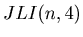, n = 1,2,..,1024} and {, n = 1,2,..,580}. Please compare these two graphs．They have the same shape, and hence these graphs seem to have Fractal behavior (self-similarity). Note thatis approximately equal to.

Graph 4.5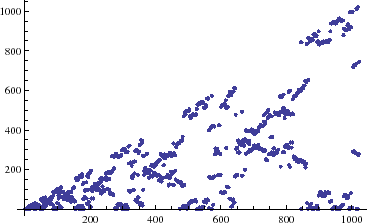Graph 4.6Example 4.5   Graph 4.7 and Graph 4.8 are the graphs of the lists {, n = 1,2,..,1024} and {, n = 1,2,..,654}. Please compare these two graphs．They have the same shape, and hence these graphs seem to have Fractal behavior (self-similarity). Note that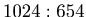is approximately equal to.

Graph 4.7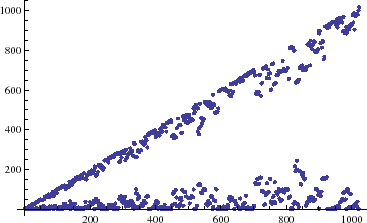Graph 4.8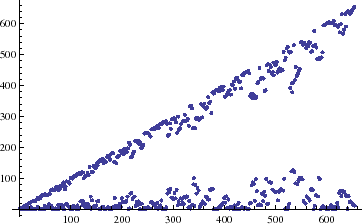Example 4.6   Graph 4.9 and Graph 4.10 are the graphs of the lists {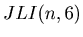, n = 1,2,..,1024} and {, n = 1,2,..,710}. Please compare these two graphs．They have the same shape, and hence these graphs seem to have Fractal behavior (self-similarity). Note thatis approximately equal to.

Graph 4.9Graph 4.10Example 4.7   Graph 4.11 and Graph 4.12 are the graphs of the lists {, n = 1,2,..,1024} and {, n = 1,2,..,751}. Please compare these two graphs．They have the same shape, and hence these graphs seem to have Fractal behavior (self-similarity). Note thatis approximately equal to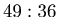.

Graph 4.11Graph 4.12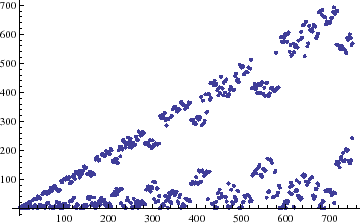Example 4.8   Graph 4.13 and Graph 4.14 are the graphs of the lists {, n = 1,2,..,1024} and {, n = 1,2,..,783}. Please compare these two graphs．They have the same shape, and hence these graphs seem to have Fractal behavior (self-similarity). Note that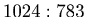is approximately equal to.

Graph 4.13Graph 4.14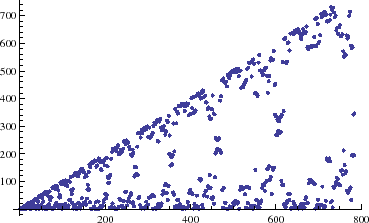Example 4.9   Graph 4.15 and Graph 4.16 are the graphs of the lists {, n = 1,2,..,1024} and {, n = 1,2,..,808}. Please compare these two graphs．They have the same shape, and hence these graphs seem to have Fractal behavior (self-similarity). Note that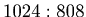is approximately equal to.

Graph 4.15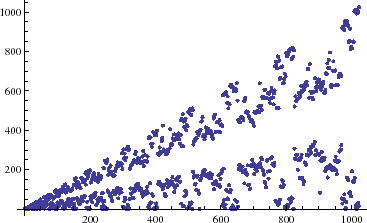Graph 4.16Example 4.10   Graph 4.17 and Graph 4.18 are the graphs of the lists {, n = 1,2,..,1024} and {, n = 1,2,..,828}. Please compare these two graphs．They have the same shape, and hence these graphs seem to have Fractal behavior (self-similarity). Note thatis approximately equal to.

Graph 4.17Graph 4.18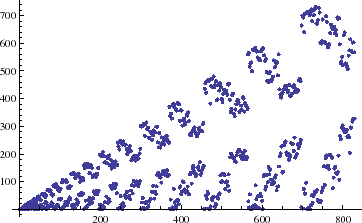Example 4.11   Graph 4.19 and Graph 4.20 are the graphs of the lists {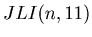, n = 1,2,..,1024} and {, n = 1,2,..,846}. Please compare these two graphs．They have the same shape, and hence these graphs seem to have Fractal behavior (self-similarity). Note thatis approximately equal to.

Graph 4.19Graph 4.20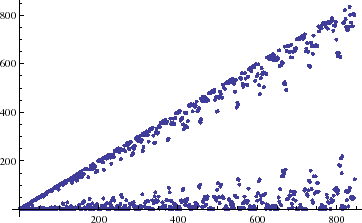: Mathematical Theory of the Josephus Problem. : The Self-Similarity of the Josephus Problems. : Linear Josephus Problem.